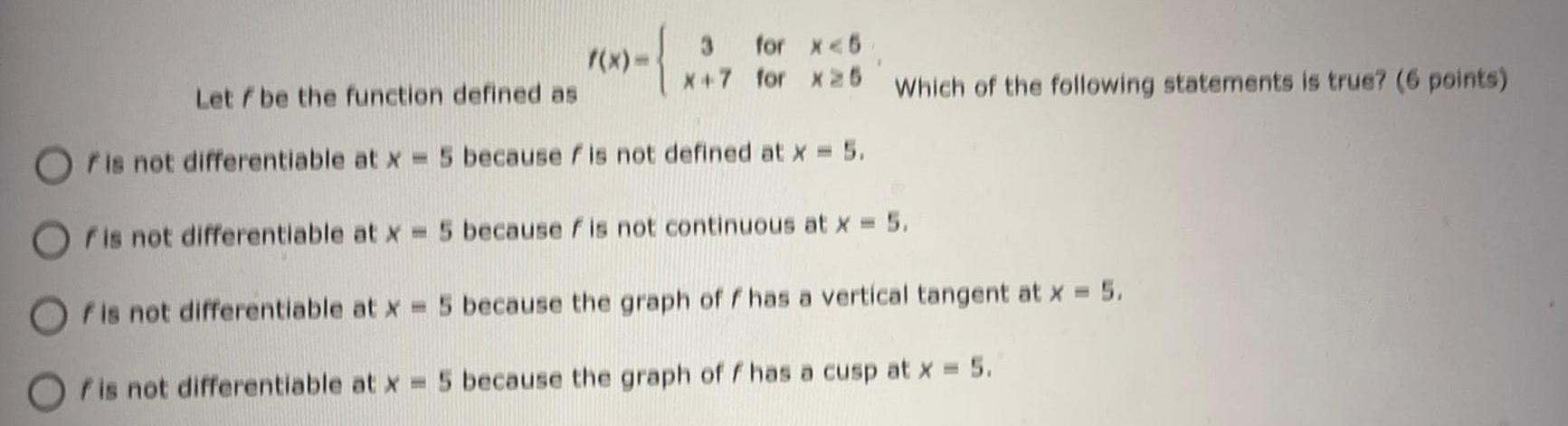Question:

# Let f be the function defined as f(x)= 3 for x<5

Last updated: 7/29/2022Let f be the function defined as f(x)= 3 for x<5 x+7 for x≥ 5 Which of the following statements is true? f is not differentiable at x = 5 because f is not defined at x = 5. f is not differentiable at x = 5 because fis not continuous at x = 5. f is not differentiable at x = 5 because the graph off has a vertical tangent at x = 5. f is not differentiable at x = 5 because the graph of f has a cusp at x = 5.